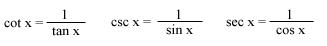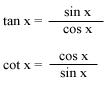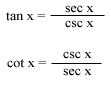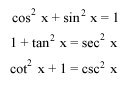Trigonometric Properties (I)

 Reciprocal Properties:Quotient Properties:Pythagorean Properties:Odd and Even Function Properties: sin(-x) = -sin x cos(-x) = cos x tan(-x) = -tan x cot(-x) = -cot x sec(-x) = sec x csc(-x) = -csc x

Back to Formula